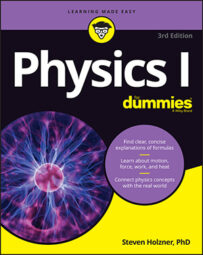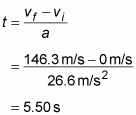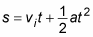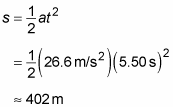##### Physics I For DummiesIn a physics equation, given a constant acceleration and the change in velocity of an object, you can figure out both the time involved and the distance traveled. For instance, imagine you’re a drag racer. Your acceleration is 26.6 meters per second2, and your final speed is 146.3 meters per second. Now find the total distance traveled. Got you, huh? “Not at all,” you say, supremely confident. “Just let me get my calculator.”

You know the acceleration and the final speed, and you want to know the total distance required to get to that speed. This problem looks like a puzzler, but if you need the time, you can always solve for it. You know the final speed, vf, and the initial speed, vi (which is zero), and you know the acceleration, a. Because vf – vi = at, you know thatNow you have the time. You still need the distance, and you can get it this way:The second term drops out because vi = 0, so all you have to do is plug in the numbers:In other words, the total distance traveled is 402 meters, or a quarter mile. Must be a quarter-mile racetrack.